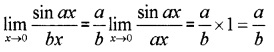# Plus One Maths Chapter Wise Questions and Answers Chapter 13 Limits and Derivatives

Students can Download Chapter 13 Limits and Derivatives Questions and Answers, Plus One Maths Chapter Wise Questions and Answers helps you to revise the complete Kerala State Syllabus and score more marks in your examinations.

## Kerala Plus One Maths Chapter Wise Questions and Answers Chapter 13 Limits and Derivatives

### Plus One Maths Limits and Derivatives Three Mark Questions and Answers

Question 1.
Evaluate the following: (3 score each)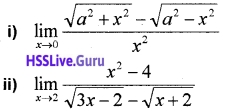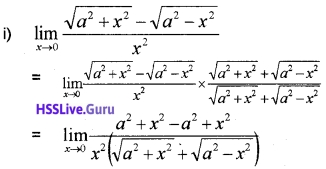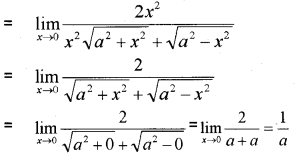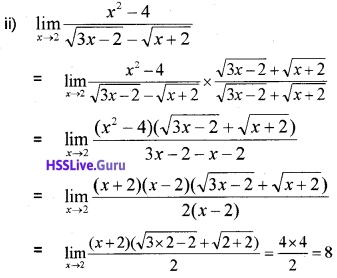Question 2.
Evaluate the following: (3 score each)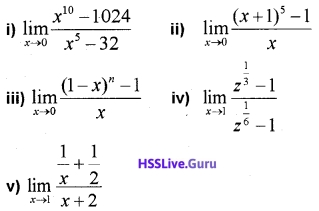ii) Put x + 1 = y, x → 0, y → 1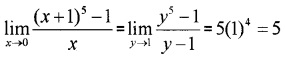iii) Put 1 – x = y, x → 0, y → 1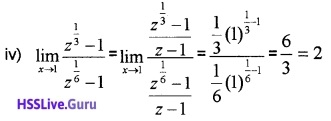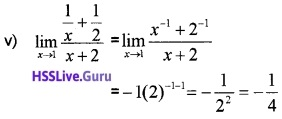Question 3.
Evaluate the following: (3 score each)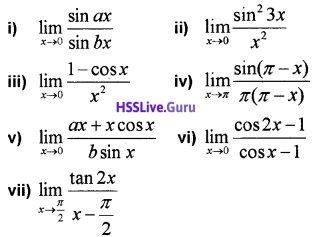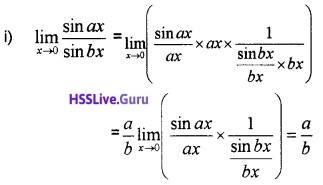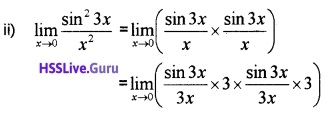= 1 × 3 × 1 × 3 = 9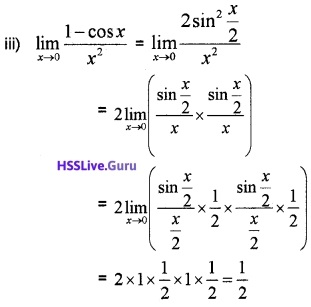iv) Put π – x = y, x → π, y → 0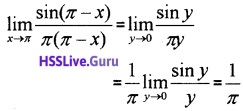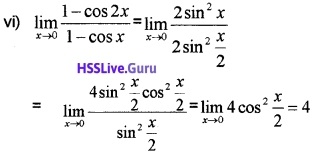vii) Put x – $$\frac{\pi}{2}$$ = y, x → $$\frac{\pi}{2}$$, y → 0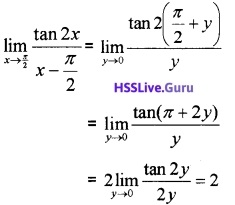Question 4.
Evaluate the following: (3 score each)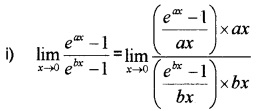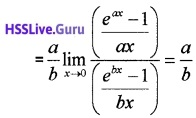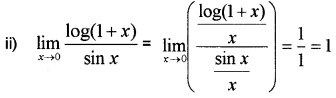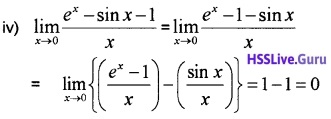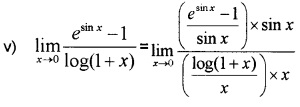= $$\frac{1}{1}$$ × 1 = 1.Question 5.
Find $$\lim _{x \rightarrow 0} f(x)$$ and $$\lim _{x \rightarrow 1}$$ f(x) where
f(x) = $$\left\{\begin{array}{cc}{2 x+3,} & {x \leq 0} \\{3(x+1),} & {x>0} \end{array}\right.$$.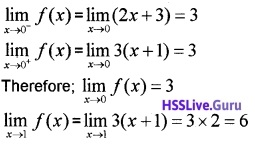Question 6.
Find $$\lim _{x \rightarrow 1}$$f(x) where f(x) = $$\left\{\begin{array}{cc} {x^{2}-1,} & {x \leq 1} \\{-x^{2}-1,} & {x>1}\end{array}\right.$$.Therefore; $$\lim _{x \rightarrow 1^{-}}$$f(x) ≠ $$\lim _{x \rightarrow 1^{-}}$$f(x)
Hence $$\lim _{x \rightarrow 1^{-}}$$f(x) does not exist.Question 7.
Evaluate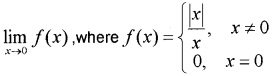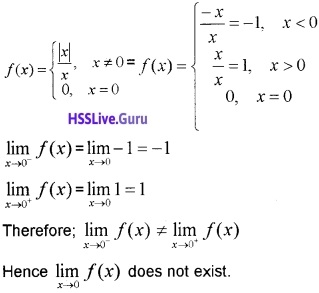### Plus One Maths Limits and Derivatives Practice Problems Questions and Answers

Question 1.
Evaluate the following:(2 score each)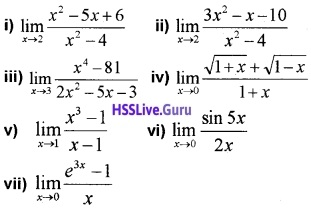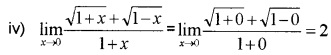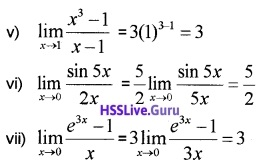Question 2.
Find the derivatives of the following: (2 score each)

1. y = (x – d)(x – b)
2. y = (ax2 + b)2
3. y = $$\frac{x-a}{x-b}$$
4. y = x-3(5 + 3x)

1.= (x – a) × 1 + (x – b) × 1
= x – a + x – b = 2x – a – b

2. y = a2x4 + b2 + 2abx2
$$\frac{d y}{d x}$$ = 4a2x3 + 4abx.

3.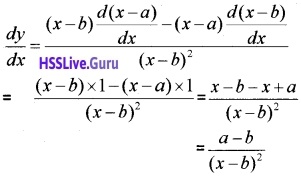4. y = 5x-3 + 3x-2
$$\frac{d y}{d x}$$ = 5(-3)x-3-1 + 3(-2)x-2-1
= -15x-4 – 6x-3Question 3.
Find the derivatives of the following: (3 score each)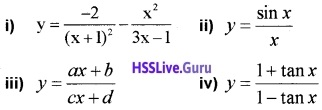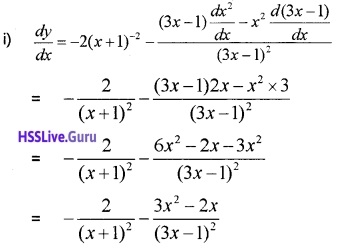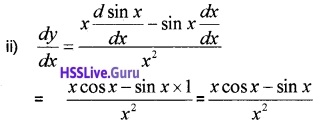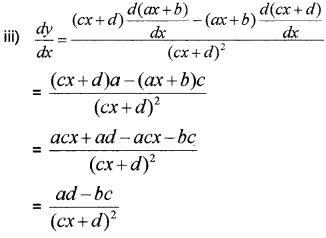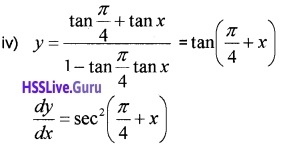Question 4.
If xy = c2 , prove that x2 $$\frac{d y}{d x}$$ + c2 = 0.
xy = c2 ⇒ y = $$\frac{c^{2}}{x}$$
Differentiating with respect to x;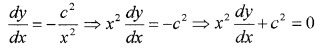Question 5.
Evaluate: $$\lim _{x \rightarrow 0} \frac{(x+5)^{2}-25}{x}$$.Question 6.
Find the derivative of f(x) = x sin x.Find the derivative of f(x) = $$\frac{\sin x}{x}$$.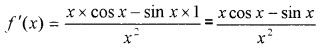Evaluate $$\lim _{x \rightarrow 0} \frac{\sin a x}{b x}$$.